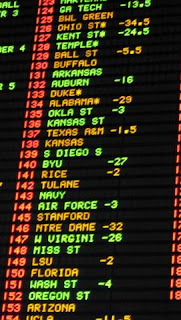## Tuesday, January 12, 2010

### The Fundamental Theorem Of Betting

I used two popular websites to get betting odds for the today's match between Egypt and Nigeria. Here are some odds from two example sites:

Site 1: Egypt (2.90) - X (3.20) - Nigeria (2.35)
Site 2: Egypt (2.65) - X (3.10)- Nigeria (2.65)Which site of the two should be preferred over the other? Generally, how to compare the attractiveness of different betting odds? In what follows, I am presenting what I call as the "fundamental theorem of betting".

Consider just the single game "Egypt vs Nigeria" which has 3 possible turnouts: "Egypt wins", "nobody wins" and "Nigeria wins". Let us also consider that we have a budget of Δ dollars and that we split this sum to all three turnouts, betting Δ1, Δ2 and Δ3 respectively. Finally, for every event, we expect to win some money, say W1, W2 and W3. In general, we are just interested in winning more money than we spent ( Δ dollars), so for every event that might take place (e.g. "Egypt wins" ) we might want W1 > Δ or W2 > Δ or W3 > Δ. But we might want to ask: Can all of these inequalities hold? The obvious answer is no. Otherwise, the booker would go bankrupt. Next, we have to ask: What values do the W's satisfy? It turns out that this is a linear function, with coefficients determined by the betting odds of the booker!

Consider that we have a budget Δ and we want to split this in all k possible turnouts. Thus, we will have to pay Δ1, Δ2,Δ3,..., and Δk respectively. For every turnout i the booker provides a betting odd Mi. This means that we will win Wi = Mi * Δi, in case of this turnout. Finally, every profit has a specific ratio of the whole budget Δ. This is the real profit since we are interested in making more money than we spent. For every turnout the profit will be denoted as: Ai = Wi / Δ. One can easily see that:

(A1/M1)+(A2/M2)+...+(Ak/Mk) = 1

For clearer inspection:This is what one could call the "Fundamental Theorem Of Betting".

Remember that the μ's are the booker's betting odds and the α's are the ratios of profit over the overall budget. For example, if we invested \$200 in total and Α1 = 2.0 then if turnout #1 happened we would win 200x 2.0 = \$400 and so on. When an α is greater than 1 this means that we won more than we invested in total and so we are interested in establishing at least one α greater than 1.0. This means that it would be useful to consider one single quantity:

M = (1/M1)+(1/M2)+(1/M3)+...+(1/Mk)

The following cases now exist:
1) M > 1.0
If this is the case then the booker is going bankrupt. Players can construct a set of α's such that all of them are greater or equal to 1.0. This means that every player either wins more than he spent in total or he gets his money back (zero loss)2) M = 1.0
In this border case, the players can set all α's to 1.0 and never suffer a loss. This is not very meaningful and players might try to disturb the ratios by increasing and decreasing some of the ratios.

3) M < 1.0
This is expected to be much more frequent in real world betting odds. Players will have to decide how to distribute their bets.

What's more important, the quantity M can be used as a metric of how attractive the betting odds are. The greater the quantity M is, the better the odds are for the players. Going back to the example in the beginning we have:

Site 1: M = (1/2.90)+(1/3.20)+(1/2.35) = 1.0828
Site 2: M = (1/2.65)+(1/3.10)+(1/2.65) = 1.0773

One can see with a single arithmetic comparison that Site 1 gives slightly better margins for profits (1.0828 is bigger) and it should be preferred to Site 2. Even within the same book house, players should prefer games with higher M factors than others. The first step to a complete "Theory of Betting" has been made!